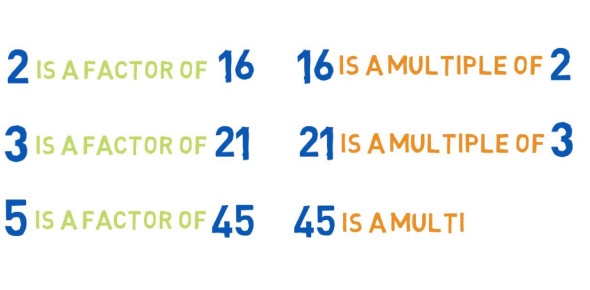# Factors And Multiples Questions! Math Trivia Quiz

9 Questions | Total Attempts: 14684Settings.

• 1.
Which number is a multiple of 6?
• A.

1

• B.

2

• C.

3

• D.

42

• 2.
Which is the greatest common factor of 45 and 60?
• A.

5

• B.

15

• C.

30

• D.

45

• 3.
Which pair of numbers has 60 as their least common multiple?
• A.

12 and 30

• B.

30 and 45

• C.

40 and 60

• D.

10 and 6

• 4.
A number is a multiple of 6 if ________________________
• A.

The last digit is 6

• B.

Its last two digits form a number divisible by 6

• C.

The sum of all of its digits is divisible by 6

• D.

The sum of all its digits is divisible by 3 and the last digit is even

• 5.
Which two numbers are relatively prime?
• A.

9 and 16

• B.

18 and 27

• C.

21 and 56

• D.

30 and 45

• 6.
How many multiples does 10 have?
• A.

4

• B.

9

• C.

100

• D.

An infinity

• 7.
Which number is a factor of 111?
• A.

3

• B.

9

• C.

11

• D.

21

• 8.
What is the greatest common factor of 12 and 18?
• A.

2

• B.

4

• C.

6

• D.

9

• 9.
Which of the following are factors of 1710?
• A.

2,4,5,10

• B.

2,5,7,10

• C.

2,3,5,10

• D.

2,3,5,8,10

Related TopicsBack to top